# Area of Triangle

Go back to  'Area'

 1 Concept Video on Area of Triangles 2 Introduction to a Triangle 3 Area of a Triangle 4 Area of a Triangle Formula 5 Tips and Tricks 6 Properties of a Triangle 7 How to Find The Area of a Triangle 8 Solved Examples on Area of Triangles 9 Practice Questions on Area of Triangles 10 Challenging Question on Area of Triangles 11 Maths Olympiad Sample Papers 12 Frequently Asked Questions (FAQs) 13 FREE Worksheets on Area of Triangles

## Learn about Area of Triangles the Cuemath Way

We understand that studying Math only through formulas can seem boring.

Our videos help you understand the concepts in a simpler, fun and quicker way.

Watch this video to see how area of triangles is taught the Cuemath Way.

## Introduction to a Triangle

Triangles are three-sided closed figures.

They can be acute, obtuse or equiangular triangles when classified based on their angles.

They can be scalene, isosceles or equilateral when classified based on their sides.

Drag the vertices in the simulation below to see the types of triangles and its elements.

## Area of a Triangle

The area of a triangle is the amount of space enclosed between the sides of the triangle.

Consider the triangle $$ABC$$

The shaded interior region enclosed within $$AB$$, $$BC$$ and $$AC$$ is the area of the given triangle.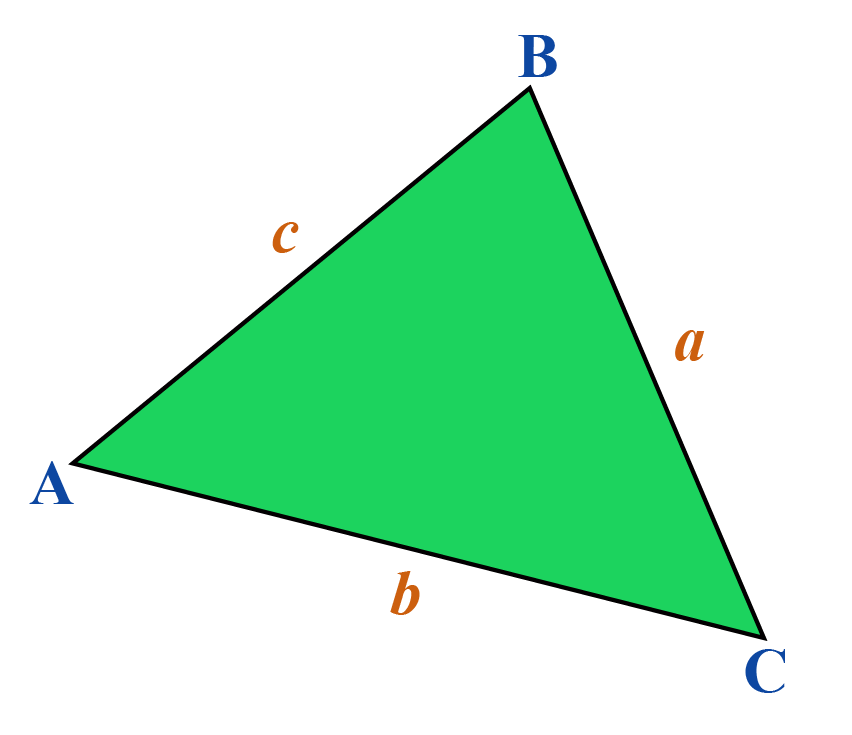## Area of a Triangle Formula

There are several ways to find the area of a triangle.

### Area of Triangle with Base and Height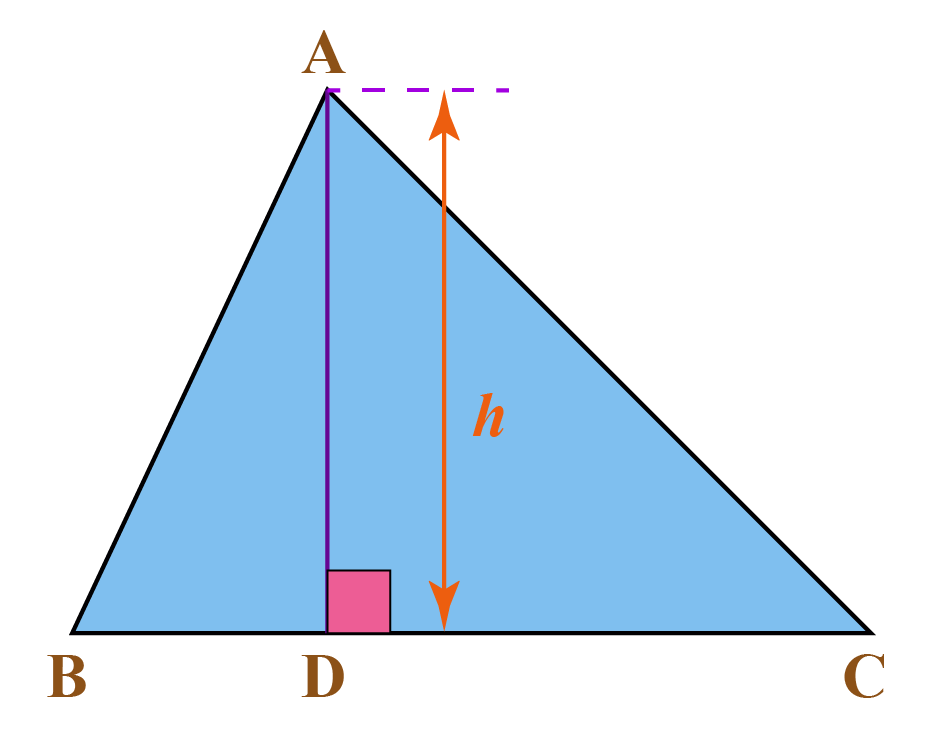In the given triangle $$ABC$$,

$$\text{Area of }\Delta ABC = \frac{1}{2} h\times \text{BC}$$

$$\text{BC}$$ is the base and $$h$$ is the height of the triangle.

 $$\frac{1}{2} \times \text{Base} \times \text{Height}$$

Use this Area of a triangle calculator to find the area when the base and height of a triangle are given.

### Area of Triangle with 3 Sides

Heron's formula is used to find the area of a triangle when the length of the 3 sides of the triangle are known.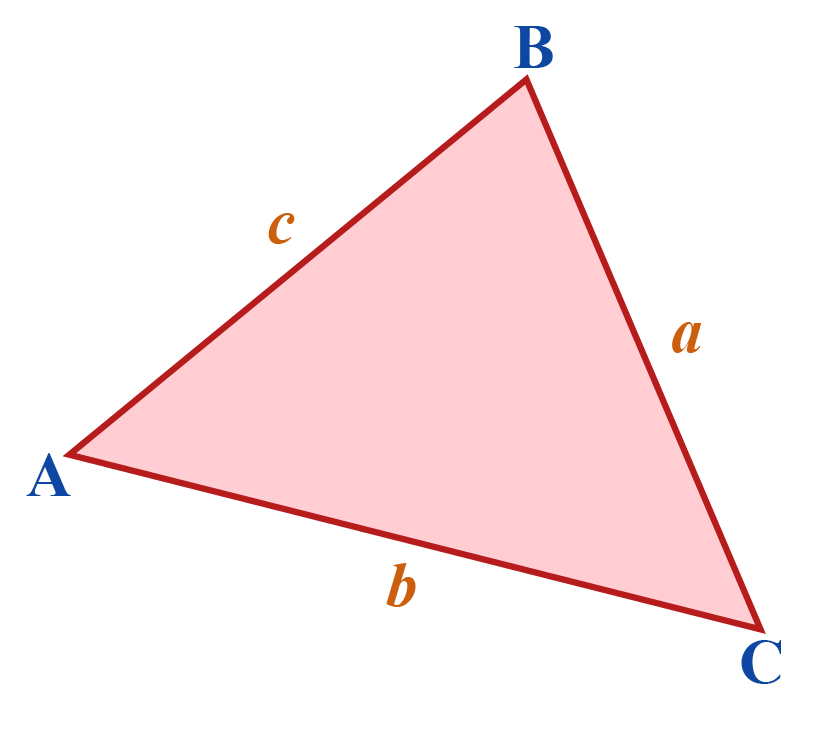Consider the triangle $$ABC$$ with sides $$a$$, $$b$$ and $$c$$.

Heron's formula to find the area of the triangle is:

 $$\sqrt {s(s - a)(s - b)(s - c)}$$

Note that $$(a + b + c)$$ is the perimeter of the triangle.

$$s$$ is the semi-perimeter which is given by:

$s = \frac{{a + b + c}}{2}$

Use this Area of a triangle calculator to find the area of a triangle using Heron's formula.

### Area of Triangle with 2 Sides and Included Angle (SAS)

There are three variations to the same formula based on which sides and included angle are given.

Consider the triangle,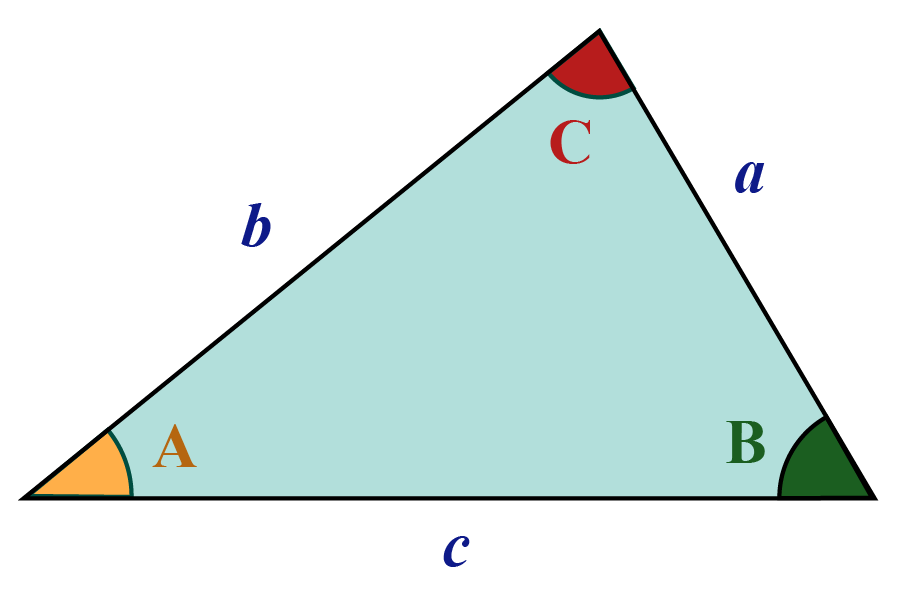When sides '$$b$$' and '$$c$$' and included angle $$A$$ is known, the area of the triangle is:

 $$\frac{1}{2} \text{bc} \times sin(A)$$

When sides '$$b$$' and '$$a$$' and included angle $$B$$ is known, the area of the triangle is:

 $$\frac{1}{2} \text{ab} \times sin(C)$$

When sides '$$a$$' and '$$c$$' and included angle $$C$$ is known, the area of the triangle is:

 $$\frac{1}{2} \text{ac} \times sin(B)$$

Use this Area of a triangle calculator to find the area when two sides and included angle is known (SAS)Tips and Tricks
1. An easy way to recall the formula for the area of a triangle when two sides and the included angle are given is to remember: 'abc'
Area of a triangle = $$\frac{1}{2}$$ab $$sin$$(C)
2. Area of an isosceles triangle and equilateral triangle can be derived from Heron's formula.

## Properties of a Triangle

• The sum of the length of the two sides of a triangle is greater than the length of the third side.
• The difference between the two sides of a triangle is less than the length of the third side.
• Angle sum property of a triangle states that the sum of all the three internal angles is $$180^\circ$$
• The external angle and the internal angle at a vertex of a triangle form a linear pair. i.e, they add up to $$180^\circ$$
• The Exterior angle property of a triangle states that the exterior angle of a triangle is equal to the sum of the interior opposite angles.
• Two triangles are said to be similar if the ratio of the corresponding sides of the two triangles are proportional.

## How to find Area of a Triangle

Area of a Triangle Formula
Base and height of a triangle are given $$\frac{1}{2} \times \text{base} \times \text{height}$$
Sides of a triangle $$(a,b,c)$$ are given $$\sqrt {s(s - a)(s - b)(s - c)}$$

where $$a, \, b, \, c$$ are the sides and $$s$$ is the semi-perimeter $$s = \frac{a+b+c}{2}$$
Two sides and the included angle are given $$\frac{1}{2}\:{side_1} \times{side_2} \times sin(θ)$$

where '$$\theta$$' is the angle between the given two sides
Area of an equilateral triangle $$\frac{\sqrt{3}}{4}\text{a}^2$$

where $$a$$ is the side of the triangle
Area of an Isosceles triangle $$\frac{1}{4}b\sqrt {4{a^2} - {b^2}}$$

where $$b$$ is the base and $$a$$ is the measure of equal side.

Use the Formulas listed above to calculate the area of a triangle based on the given parameters.

The area of a triangle calculator will help you find them easily.

## Area of a Triangle - Solved Examples

 Example 1

Deduce the area of an equilateral triangle from Heron's formula and find the area of an equilateral triangle of side 10 cm.

Solution:

We know that Herons formula is $$\sqrt {s(s - a)(s - b)(s - c)}$$ where $$a$$, $$b$$ and $$c$$ are sides of the triangle and $$s$$ is the semi-perimeter.

In an equilateral triangle, all sides are equal.

Therefore, $$a = b = c$$

For our convenience, let us use $$a$$

Semi-perimeter \begin{align} s = \frac{3a}{2} \end{align}

\begin{align} Area \!&=\!\sqrt{ \frac{3a}{2} (\frac{3a}{2}\! -\! a)(\frac{3a}{2}\! - \!a)(\frac{3a}{2}\! -\! a)} \\ &=\sqrt{ \frac{3a}{2}(\frac{a}{2} )(\frac{a}{2})(\frac{a}{2})} \\ &= \sqrt{\frac{3a^4}{16}} \\ &=\frac{\sqrt{3}}{4}a^2 \end{align}

Substituting the value $$a = 10 cm$$, we get:

\begin{align} \frac{\sqrt{3}}{4}10^2 &\approx 43.30 \:\text{cm}^2 \end{align}

Area of an equilateral triangle of side 10 cm is

 Area of equilateral triangle $$\approx 43.30 \,cm$$
 Example 2

The signboard at a cross road needs to be repainted.

The measurements are as given in the image.

Find the area to be painted.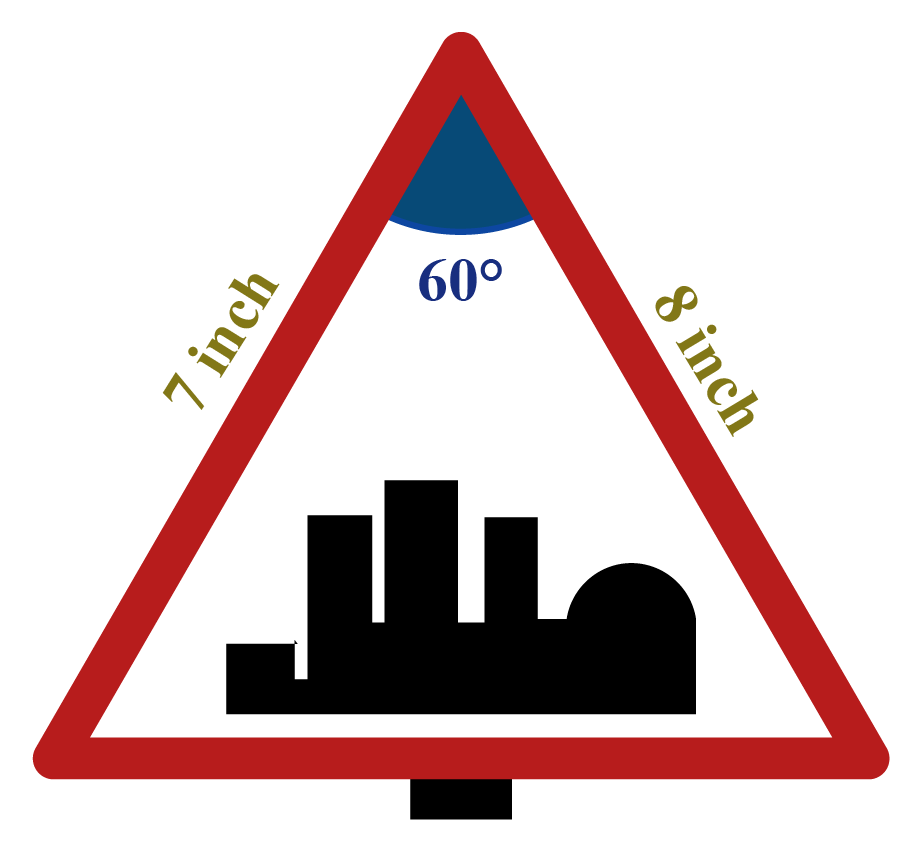Solution:

The area of a triangle when 2 sides and included angle are given is:

\begin{align} &\frac{1}{2}\:{side_1} \times{side_2} \times sin(θ) \\ &=\frac{1}{2} \times 7 \times 8 \times \frac{\sqrt{3}}{2} (\because sin \:60 = \frac{\sqrt{3}}{2}) \\ &=24.24 \end{align}

 Area to be painted $$= 24.24 \:inch^2$$
 Example 3

The sides of a triangular park are in the ratio $$12:17:25,$$ and its perimeter is $$1080 \; m$$

What is its area?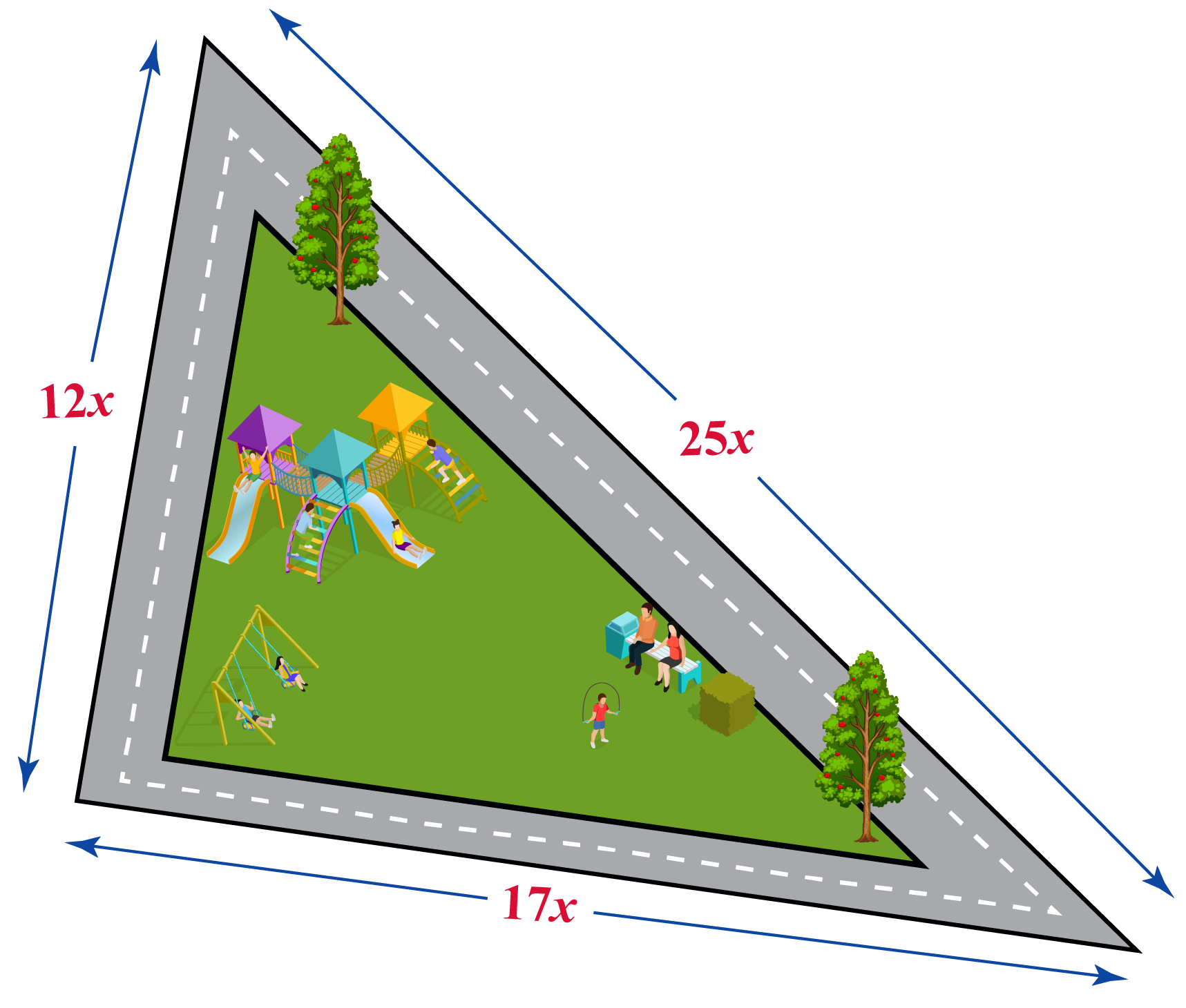Solution:

The sides of the triangle are $$12x$$, $$17x$$ and $$25x$$

\begin{align}12x + 17x + 25x &= 1080\\ \Rightarrow 54x &= 1080\\ \Rightarrow x &= 20\end{align}

Thus, the sides of the triangle are:

$240 m, \; 340 m,\; 500 m$

Now, the semi-perimeter of the triangle is:

$s = \frac{{1080}}{2} = 540\:m$

Using Heron’s Formula, the area of the triangle is:

\begin{align}&A\! =\! \sqrt {540\left( {540 \!- \!240} \right)\left( {540\! - \!340} \right)\left( {540\! -\! 500} \right)} \\ &\quad= \sqrt {540 \times 300 \times 200 \times 40} \\& \quad= 36,000\, \rm{m^2}\end{align}

 Area of the triangular park $$= 36,000 \:m^2$$
 Example 4

Find the area of the shaded region in the following figure.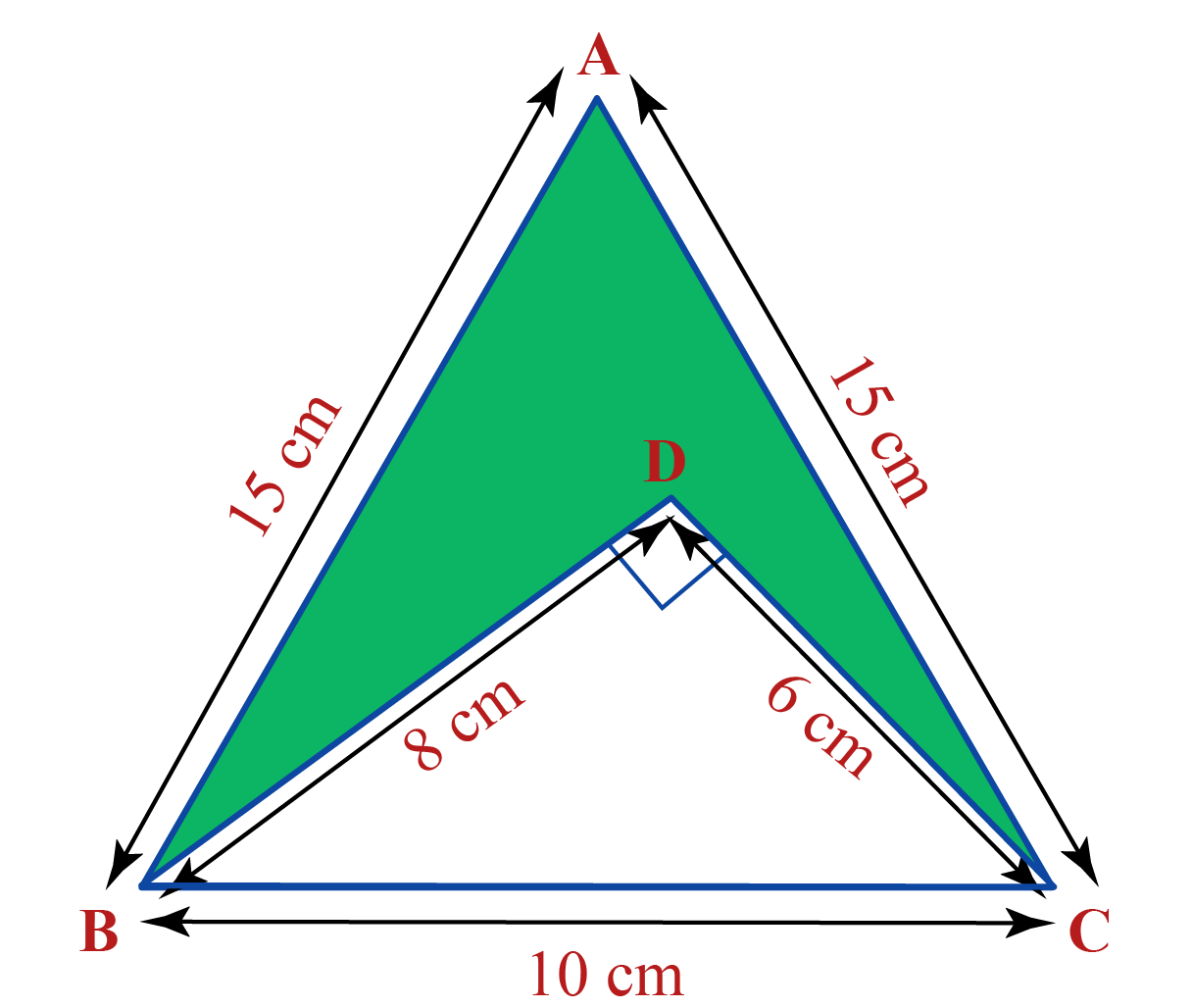Solution:

The altitude for $$\Delta ABC$$ is:

\begin{align} &h \;= \sqrt {{{15}^2} - {5^2}} \;(\text{by Pythagorean theorem})\\ &\quad = 10\sqrt 2\, \rm{cm} \end{align}

Thus,

\begin{align}&Area\left( {\Delta ABC} \right) = \frac{1}{2} \times BC \times h\\& \qquad\qquad\qquad\;= \frac{1}{2} \times 10 \times 10\sqrt 2 \\&\qquad\qquad\qquad\;= 50\sqrt 2 \,\rm{cm^2}\end{align}

Also,

\begin{align}&area\left( {\Delta BDC} \right) = \frac{1}{2} \times CD \times BD\\&\qquad\qquad\qquad\; = \frac{1}{2} \times 6 \times 8 = 24\, \rm{cm^2}\end{align}

Thus, the area of the shaded region is:

\begin{align} & A \;= area(\Delta ABC) - area(\Delta BDC)\\ &\quad = (50\sqrt 2 - 24)\,\rm{cm^2}\\ &\quad \approx 46.7\, \rm{cm^2} \end{align}

 Area of the shaded region $$= 46.7\:\text{cm}^2$$
 Example 5

Find the area and perimeter of an equilateral triangle $$\Delta ABC$$ whose height is $$10 cm$$

Solution:

Let the length of each of the triangle’s sides be '$$a$$' units.

Consider the following figure: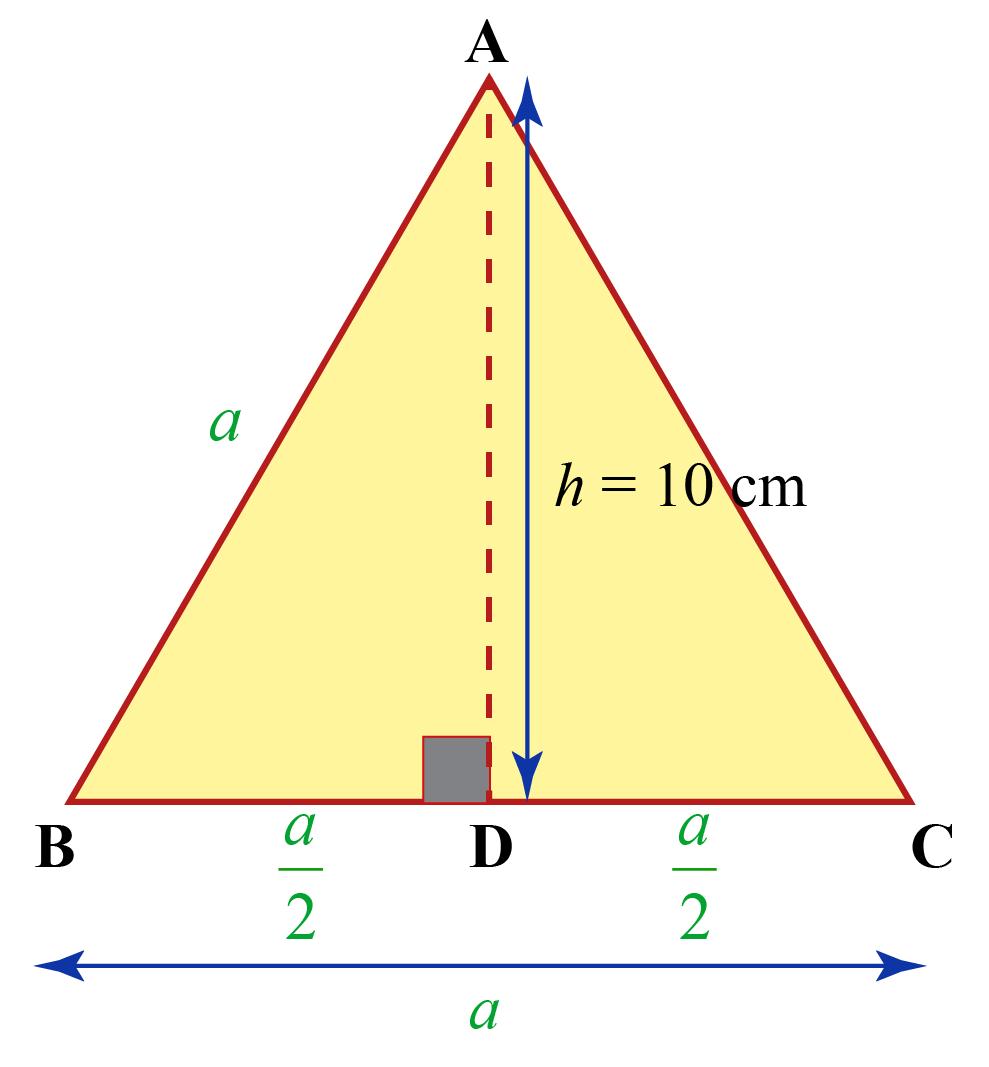We have in $$\Delta ABD$$:

\begin{align}&{a^2}\! \!=\! {h^2}\! \!+\! \!{\left( {\frac{a}{2}} \!\right)^2}\;(\text{by Pythagoras theorem}\!)\\& \Rightarrow \frac{{3{a^2}}}{4} = {h^2}\\& \Rightarrow a = \frac{{2h}}{{\sqrt 3 }}\end{align}

Area of $$\Delta ABC$$ is

\begin{align}&A = \frac{{\sqrt 3 }}{4}{a^2} = \frac{{\sqrt 3 }}{4} \times \frac{{4{h^2}}}{3}\\&\frac{{{h^2}}}{{\sqrt 3 }} = \frac{{100}}{{\sqrt 3 }}cm \approx ~57.7 \,\rm{cm^2}\end{align}

 Area of given triangle $$= 57.7\:\text{cm}^2$$

Perimeter of $$\Delta ABC$$

\begin{align}&P = 3a = 3 \times \frac{{2h}}{{\sqrt 3 }}\\&\quad = 2\sqrt 3 h = 20\sqrt 3 cm \approx 34.6\, \rm{cm}\end{align}

 Perimeter of given triangle $$= 34.6 \:\text{cm}$$

## Practice Questions

Here are few questions for you to practice. Select/Type your answer and click the "Check Answer" button to see the result.

Area of a triangle worksheet is available at the end of the page for you to try and solve!Challenging Question
1. In the given figure, $$ABCD$$ is a parallelogram.

If the area of $$\Delta BFC = 40\;cm^2$$, calculate the area of $$\Delta ABE$$.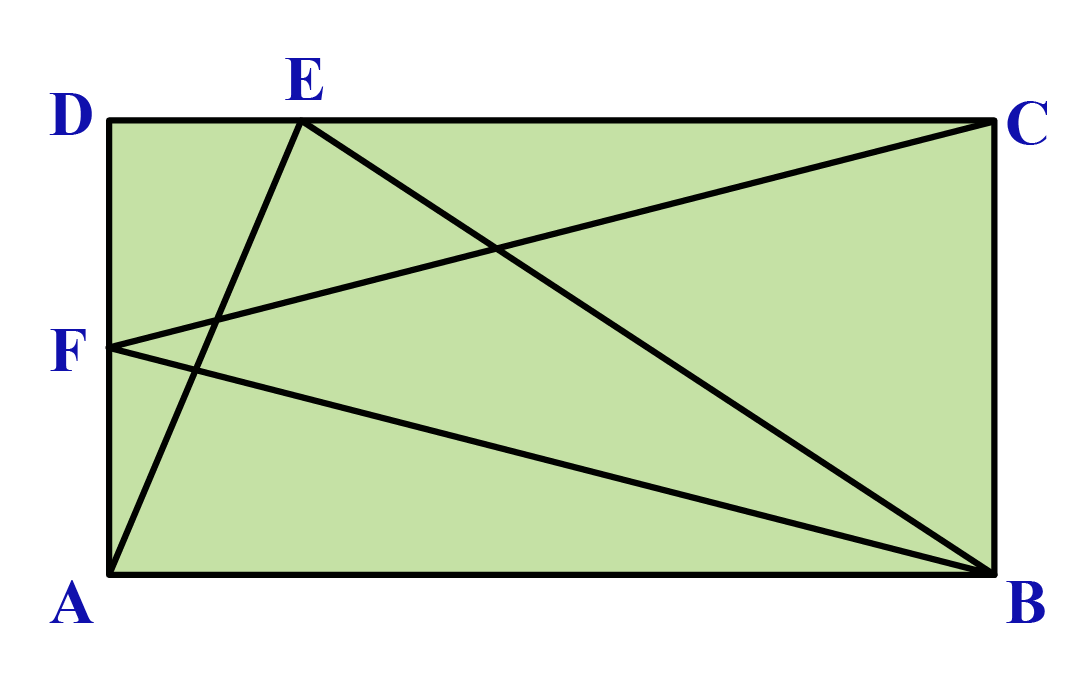IMO (International Maths Olympiad) is a competitive exam in Mathematics conducted annually for school students. It encourages children to develop their math solving skills from a competition perspective.

## 1. What is the area of a triangle?

The area of a triangle is the amount of space or region enclosed between the sides of the triangle.

## 2. What is the area when two sides of a triangle and included angle are given?

The area of a triangle when two sides $$a$$ and $$b$$ and the included angle $$\angle C)$$ is $$\frac{1}{2}ab\:sin (C)$$.

## 3. How to calculate the area of a triangle?

Use the formulas from the section How to Find the Area of a Triangle to calculate the area.

Scroll up and use the calculator as well.

Mensuration
grade 9 | Questions Set 1
Mensuration
Mensuration